### Home > CAAC > Chapter 8 > Lesson 8.3.1 > Problem8-100

8-100.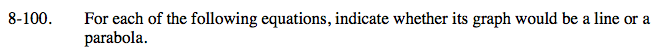A line can be written in y = mx + b form.

A parabola has an x2 in the equation.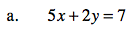Solve the equation for y.
If it is a line, it will be in y = mx + b form.

It is a line.
5x + 2y = 7

$y=-\frac{5}{2}x+\frac{7}{2}$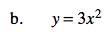It is a parabola.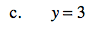It is a line.
y = 3 is the same as y = 0x + 3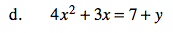It is a parabola.